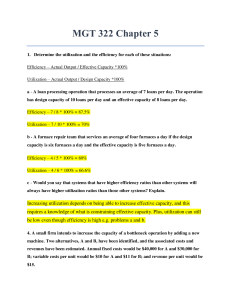# MGT 322 Chapter 5. Questions and Answers.

PLDZ-54 In Stock
\$ 11.00 USD
Description

CLICK ON THE PRODUCT INFORMATION URL

MGT 322 Chapter 5 1. Determine the utilization and the efficiency for each of these situations: Efficiency – Actual Output / Effective Capacity *100% Utilization – Actual Output / Design Capac ity *100% a - A loan processing operation that processes an average of 7 loans per day. The operation has design capacity of 10 loans per day and an effective capacity of 8 loans per day. b - A furnace repair team that services an average of four furnaces a day if the design capacity is six furnaces a day and the effective capacity is five furnaces a day. ? c - Would you say that systems that have higher efficiency ratios than other systems will always have higher utilization ratios than those other systems? Explain. 4. A small firm intends to increase the capacity of a bottleneck operation by adding a new machine. Two alternatives, A and B, have been identified, and the associated costs and revenues have been estimated. Annual fixed costs would be \$40,000 for A and \$30,000 for B; variable costs per unit would be \$10 for A and \$11 for B; and revenue per unit would be \$15. ? a - Determine each alternative’s break-even point in units.? b - At what volume of output would the two alternatives yield the same profit?? c - If expected annual demand is 12,000 units, which alternative would yield the higher profit? ? 8a. A manager is trying to decide whether to purchase a certain part or to have it produced internally. Internal production could use either of two processes. One would entail a variable cost of \$17 per unit and an annual fixed cost of \$200,000; the other would entail a variable cost of \$14 per unit and an annual fixed cost of \$240,000. Three vendors are willing to provide the part. Vendor A has a price of \$20 per unit for any volume up to 30,000 units. Vendor B has a price of \$22 per unit for demand of 1,000 units or less, and \$18 per unit for larger quantities. Vendor C offers a price of \$21 per unit for the first 1,000 units, and \$19 per unit for additional units. ? a - If the manager anticipates an annual volume of 10,000 units, which alternative would be best from a cost standpoint? For 20,000 units, which alternative would be best? ? 14. The following diagram describes a process that consists of eight separate operations, with sequential relationships and capacities (units per hour) as shown. a - What is the current capacity of the entire process?? b. If you could increase the capacity of only two operations through process improvement efforts, which two operations would you select, how much additional capacity would you strive for in each of those operations, and what would the resulting capacity of the entire process be? Show Less

Recent Reviews Write a Review
0 0 0 0 reviews